C语言重点——指针篇（一文让你完全搞懂指针）| 从内存理解指针 | 指针完全解析

C语言最核心的知识就是指针，所以，这一篇的文章主题是「指针与内存模型」

一、内存本质

「指针存储的是变量的内存地址」这句话应该任何讲 C 语言的书都会提到吧。

1.1 内存编址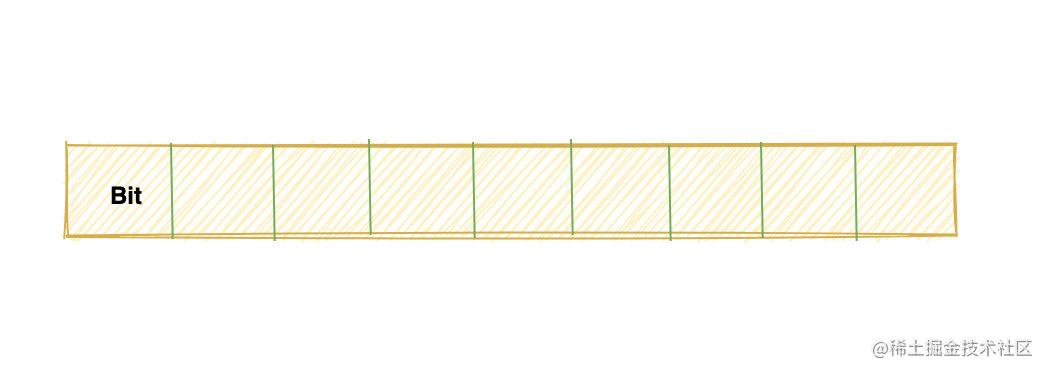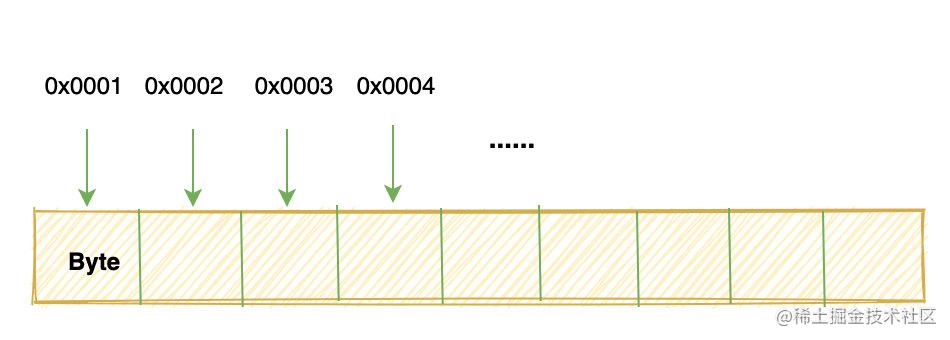1.3 变量的本质

int a = 999;
char c = 'c';

999 换算成补码就是：0000 0011 1110 0111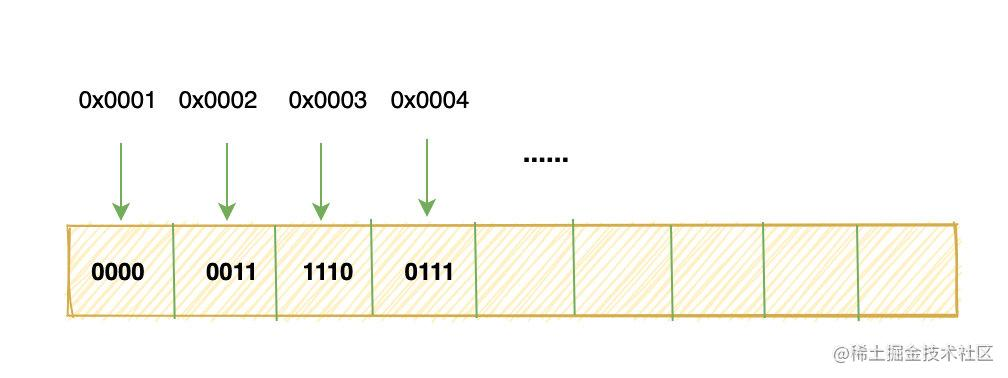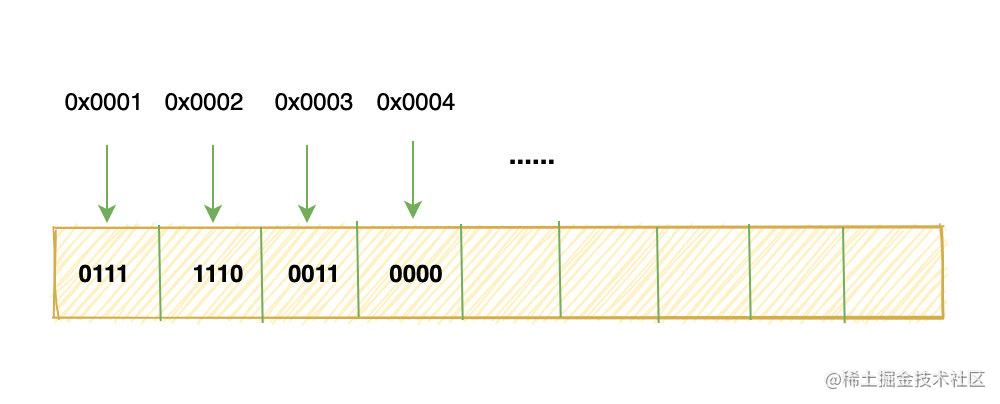二、指针是什么东西？

2.1 变量放在哪？

(PS: 实际上这个地址是虚拟地址，并不是真正物理内存上的地址

printf("%x", &a);

2.2 指针本质

int *pa = &a;

pa 中存储的就是变量 a 的地址，也叫做指向 a 的指针。

....

int func(...) {
...
};

int main() {
int a;
func(...);
};

....
};

int main() {
int a;
func(&a);
};

2.3 解引用

pa中存储的是a变量的内存地址，那如何通过地址去获取a的值呢？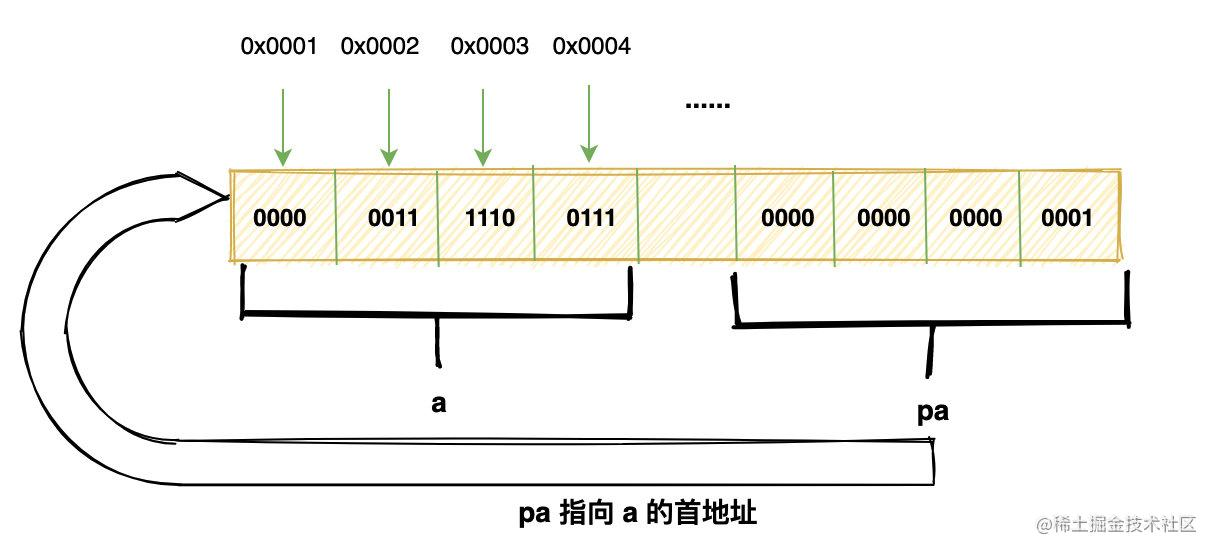pa 指针首先是一个变量，它本身也占据一块内存，这块内存里存放的就是 a 变量的首地址。

2.4 活学活用

float f = 1.0;
short c = *(short*)&f;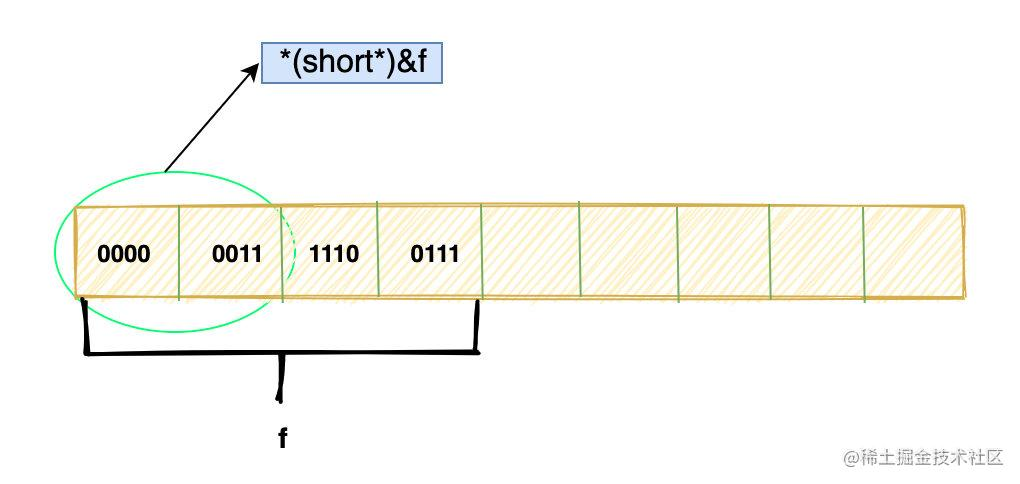1. &f取得 f 的首地址
2. (short*)&f

“噢，我认为f这个地址放的是一个 short 类型的变量”

short c = 1;
float f = *(float*)&c;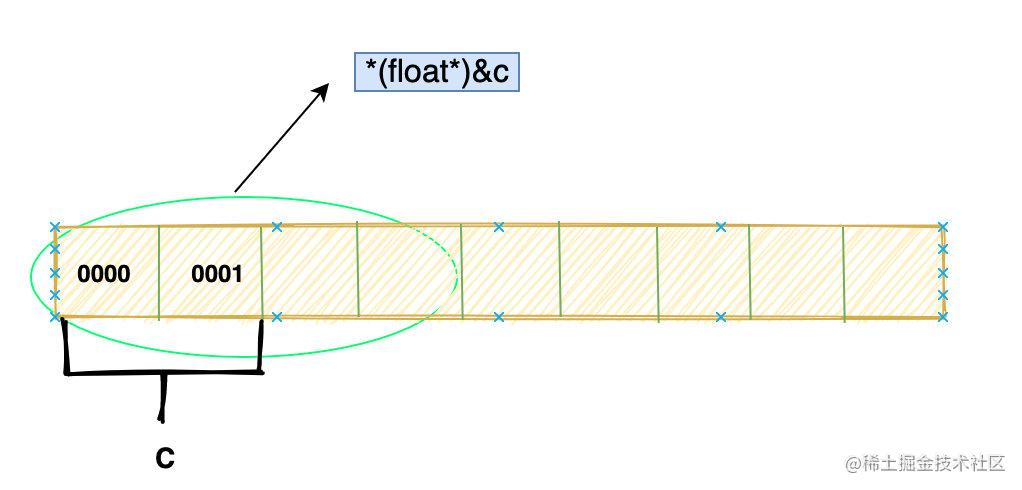(float*)&c会让我们从c 的首地址开始取四个字节，然后按照 float 的编码方式去解释。

*(float*)&c = 1.0;

2.6 看个小问题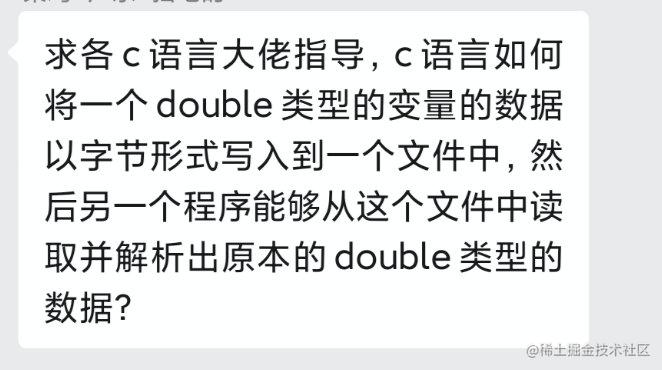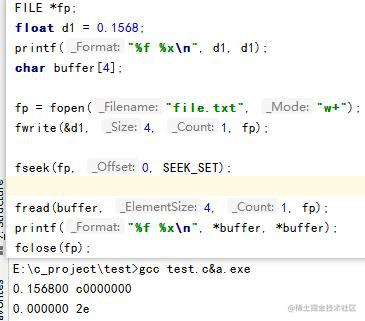char buffer;
...
printf("%f %x\n", *buffer, *buffer);

“哦，buffer 是 char类型的指针，那我取第一个字节出来就好了”。

printf("%f %x\n", *(float*)buffer, *(float*)buffer);

buffer 指向的这个地方，我放的是一个 float，你给我按照 float 去解释”

三、 结构体和指针

struct fraction {
int num; // 整数部分
int denom; // 小数部分
};

struct fraction fp;
fp.num = 10;
fp.denom = 2;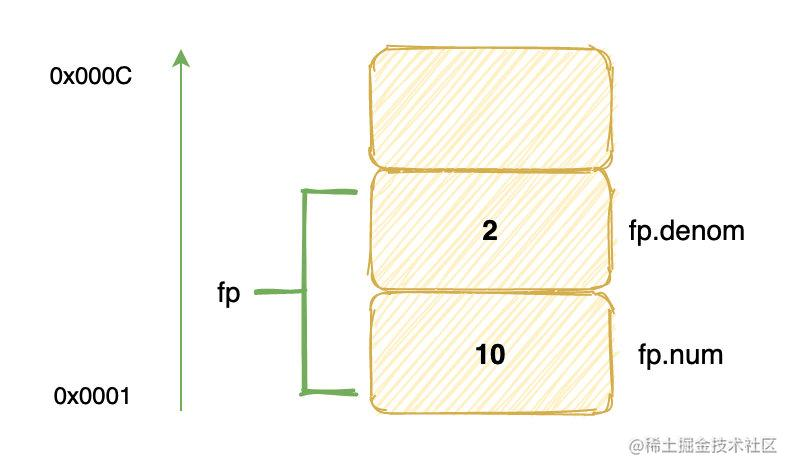((fraction*)(&fp.denom))->num = 5;
((fraction*)(&fp.denom))->denom = 12;
printf("%d\n", fp.denom); // 输出多少？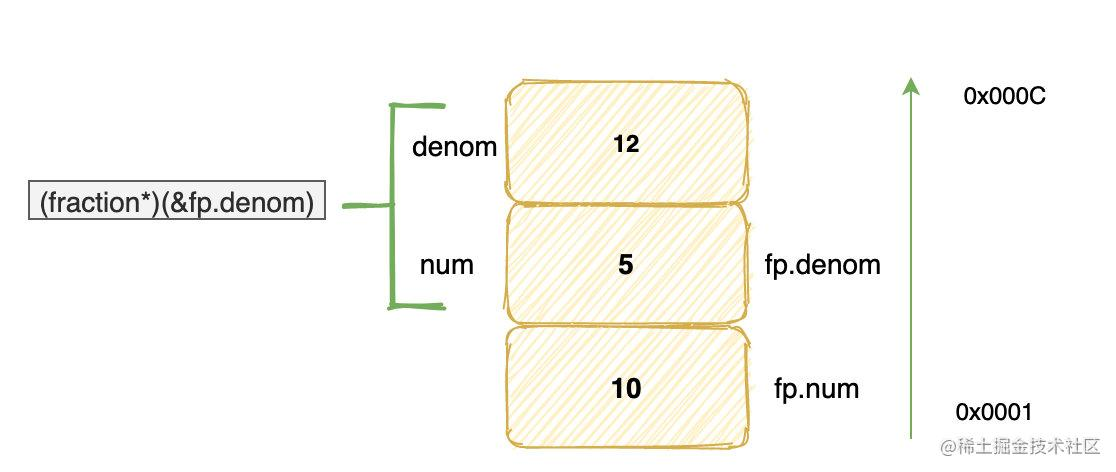((fraction*)(&fp.denom))->num = 5

((fraction*)(&fp.denom))->denom = 12

四、多级指针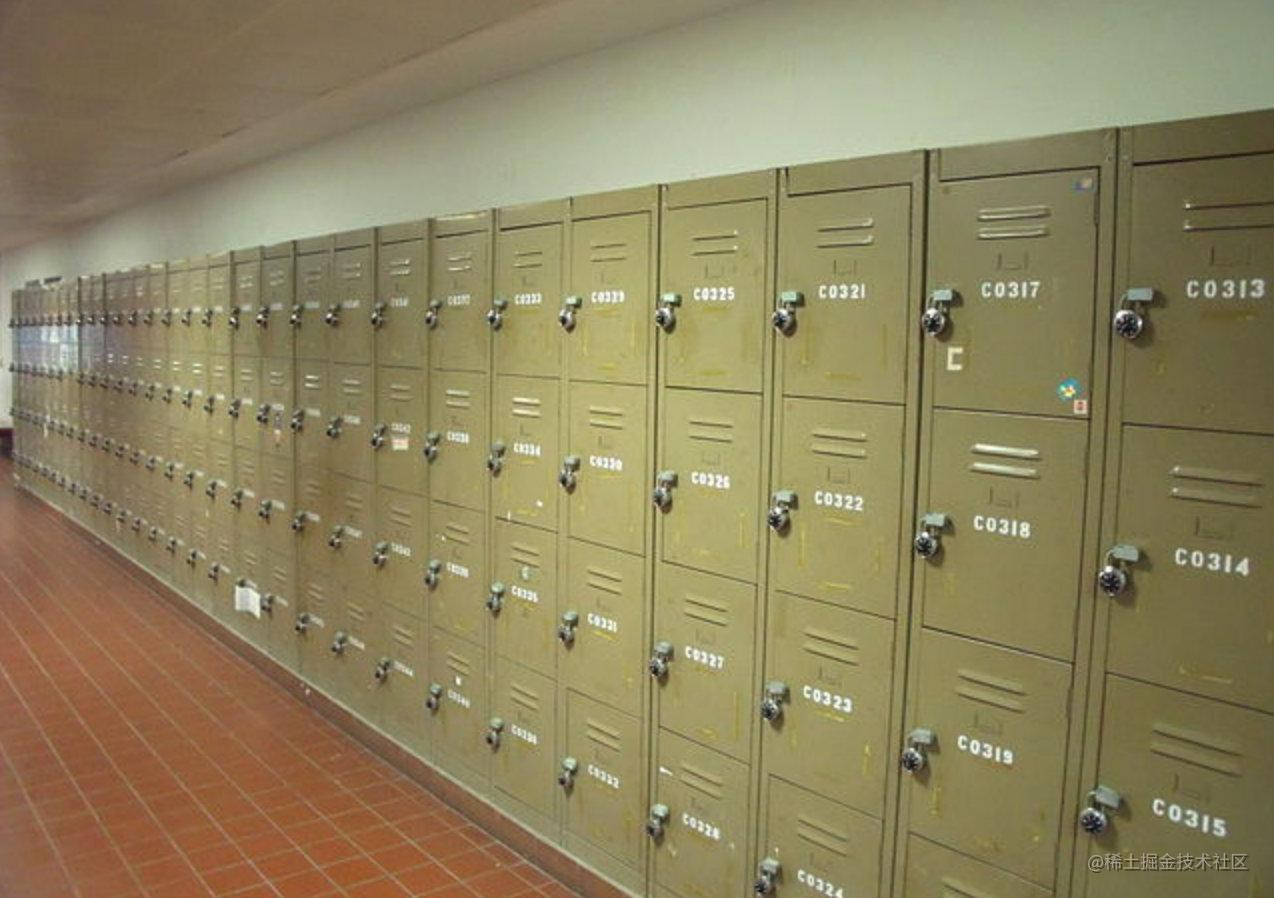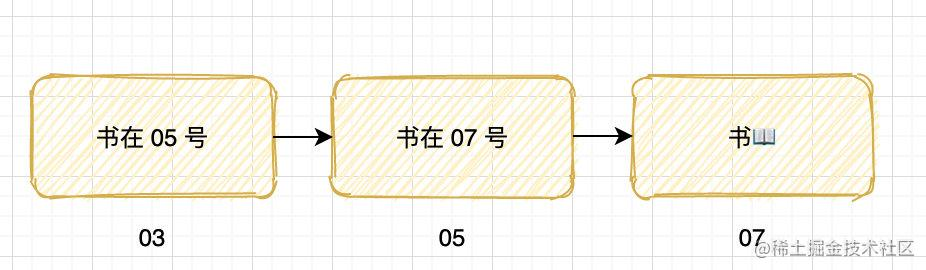int a;
int *pa = &a;
int **ppa = &pa;
int ***pppa = &ppa;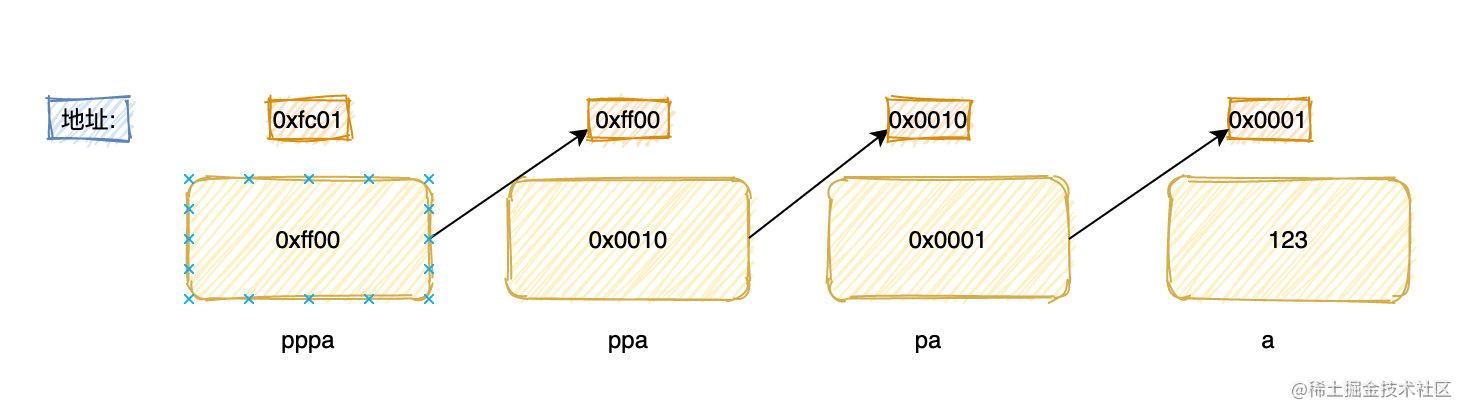• 指针本身也是一个变量，需要内存去存储，指针也有自己的地址
• 指针内存存储的是它所指向变量的地址

int ** a 可以把它分为两部分看，即 int**a，后面 *a 中的*表示 a 是一个指针变量，前面的 int* 表示指针变量 a

五、指针与数组

5.1 一维数组

int array = {10, 9, 8, 7};
printf("%d\n", *array);  // 	输出 10
printf("%d\n", array);  // 输出 10

printf("%d\n", array);  // 输出 9
printf("%d\n", *(array+1)); // 输出 9

int *pa = array;
printf("%d\n", *pa);  // 	输出 10
printf("%d\n", pa);  // 输出 10

printf("%d\n", pa);  // 输出 9
printf("%d\n", *(pa+1)); // 输出 9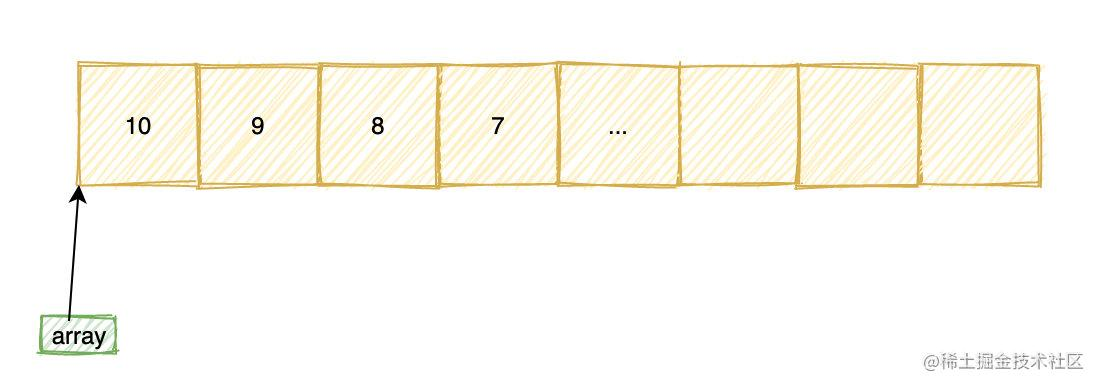printf("%u", sizeof(array));
printf("%u", sizeof(pa));

sizeof 是一个操作符，不是函数，使用 sizeof 时可以从这张表格中查询到符号的长度。

pa 仅仅是一个指向 int 类型的指针，编译器根本不知道它指向的是一个整数，还是一堆整数。

5.2 二维数组

int array = {{1, 2，3}, {4, 5，6}，{7, 8, 9}};
array = 5;

1		2		3
4		5		6
7		8		9

1		2		3		4		5		6		7		8		9

int array[n][m]

六、神奇的 void 指针

void func();
int func1(void);

void *ptr;

void 指针最大的用处就是在 C 语言中实现泛型编程，因为任何指针都可以被赋给 void 指针，void 指针也可以被转换回原来的指针类型， 并且这个过程指针实际所指向的地址并不会发生变化。

int num;
int *pi = &num;
void* pv = pi;
pi = (int*) pv;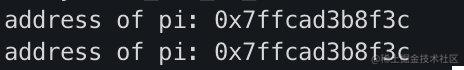void qsort(void *base,int nelem,int width,int (*fcmp)(const void *,const void *));

void 还可以用来实现 C 语言中的多态，这是一个挺好玩的东西。

• 不能对 void 指针解引用

int num;
void *pv = (void*)&num;
*pv = 4; // 错误

七、花式秀技

• 二维数组和二维指针
• 数组指针和指针数组
• 指针运算
• 函数指针
• 动态内存分配: malloc 和 free
• 堆、栈
• 函数参数传递方式
• 内存泄露
• 数组退化成指针
• const 修饰指针
• ...